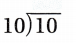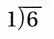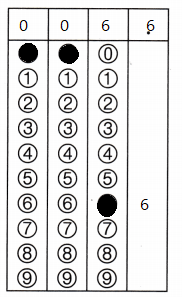Refer to our Texas Go Math Grade 3 Answer Key Pdf to score good marks in the exams. Test yourself by practicing the problems from Texas Go Math Grade 3 Module 11 Assessment Answer Key.

Choose the best term from the box to complete the sentence.

Question 1.
A number is ___ quotient is a counting number and the number can be divided into equal groups. (p. 352)
Explanation:
possible to divide or separate Nine is divisible by three.

Question 2.
___ are a set of related multiplication and division equations. (p. 339)
Explanation:
The inverse of multiplication is division.
If you multiply by a given number and then divide by the same number,
you will arrive at the same number you started with.

Concepts and Skills

Complete the related facts. TEKS 3.4.F, 3.4.J, 3.5.B

Question 3.
4 × 8 = ___
8 × __ = 32
32 ÷ __ = 8
32 ÷ 8 = __
4 × 8 = 32
8 × 4 = 32
32 ÷ 4 = 8
32 ÷ 8 = 4
Explanation:
The equations are related to inverse operation

Question 4.
3 × __ = 9
__ ÷ 3 = 3
3 × 3 = 9
9 ÷ 3 = 3
Explanation:
The equations are related to inverse operation

Question 5.
6 × __ = 42
7 × 6 = ___
___ ÷ 6 = 7
42 ÷ __ = 6
6 × 7 = 42
7 × 6 = 42
42 ÷ 6 = 7
42 ÷ 7 = 6
Explanation:
The equations are related to inverse operation

Find the quotient. TEKS 3.4.H, 3.5.B

Question 6.
2 ÷ 1 = ___
Explanation:
When a number is divided by 1 the number does not change

Question 7.
0 ÷ 7 = ___
Explanation:
So zero divided by zero is undefined. .

Question 8.Explanation:
When a number is divided by itself the answer is 1

Question 9.Explanation:
When a number is divided by  1 the answer will not change

Tell if the product will be odd or even. TEKS 3.4.I

Question 10.
8 × 22
Explanation:
176 is a even number
As the end numbers are even the answer is also even

461 × 10
Explanation:
4610 is a even number
As the end numbers are even the answer is also even

Question 12.
433 × 267
Explanation:
As the end numbers are odd the answer is also odd
176 is a even number

Question 13.
944 × 1,275
Explanation:
When even is multiplied with odd the answer will be even

Use the divisibility rule to tell if the number is odd or even. TEKS 3.4.I

Question 14.
43
Explanation:
Divisibility rule of 43 is itself and 1

Question 15.
596
Explanation:
576 the last digit is 6 is divided by 2
It is even

Question 16.
3,718
Explanation:
3718 the last digit is 8 is divided by 2
It is even

Question 17.
2,609
Explanation:
2609 the last digit is 9 is divided by 3
so, it is odd

Texas Test Prep

Fill in the bubble for the correct answer choice.

Question 18.
A pet shop has 18 hamsters. They sold 10 and put the remaining hamsters into 8 cages. How many hamsters are in each cage? TEKS 3.4.H, 3.4.K, 3.5.B
(A) 4
(B) 8
(C) 2
(D) 1
Explanation:
1 hamsters are in each cage

Question 19.
Which division equation belongs to this set of related facts? TEKS 3.4.F, 3.4.J, 3.5.B
4 × 6 = 24 6 × 4 = 24
(A) 20 ÷ 4 = 5
(B) 24 ÷ 6 = 4
(C) 18 ÷ 6 = 3
(D) 24 ÷ 3 = 8
Explanation:
24 ÷ 6 = 4 is equation belongs to this set of related facts

Question 20.
Jeremy is thinking of a 2-digit number that is divisible by 2. What could Jeremy’s number be? TEKS 3.4.1
(A) 210
(B) 63
(C) 49
(D) 78
Explanation:
78 is the 2 digit number that is divisible by 2

Question 21.
Jill has 6 puppies. The puppies play in 1 crate. How many puppies are in the crate? TEKS 3.4.H, 3.4.K, 3.5.BRecord your answer and fill in the bubbles on the grid. Be sure to use the correct place value.